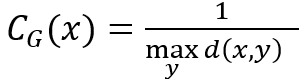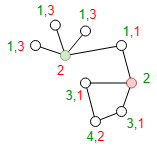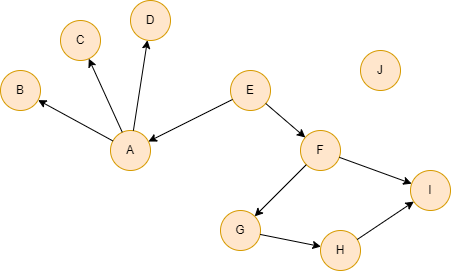# Change Nickname

Current Nickname:

Certifications

Certificate Issued at Valid until Serial No. File
Serial No. Valid until

Not having one? Apply now! >>>

Invoice

ProductName CreateTime ID Price File
ProductName CreateTime ID Price File

# Graph Centrality

## Overview

Graph centrality is used to measure the maximum shortest distance from node to other nodes in its connected component. This concept, along with other concepts (such as closeness centrality, graph diameter, etc.), can be considered jointly to determine whether a node is literally located at the very center of the graph.

The range of values of Graph Centrality is [0,1]; the larger the value, the closer the node is to the center.

## Basic Concept

### Graph Centrality

Graph centrality is defined as the reciprocal of the maximum shortest distance from a node to other nodes in its connected component. Please read the chapter Closeness Centrality for the introduction to the Shortest Distance.where `x` is the node to be calculated, `y` is any node (`x` is excluded) in the connected component that `x` is in, `d(x,y)` is the shortest distance from `x` to `y`.In the graph above, the shortest distances from each node to the red and green nodes have been marked, and the graph centrality of the red and green nodes are 0.3333 and 0.25. If only calculates the closeness centrality of the two nodes - the red node is `8/(3+3+3+2+1+1+2+1)=0.5`, the green node is `8/(1+1+1+1+2+3+4+3)=0.5` - the two values are the same; thus when closeness centrality is the same, graph centrality can serve as a subsidiary basis to determine which node is closer to the center.

## Special Case

### Lonely Node, Disconnected Graph

Lonely node is not connected with any other node, so its graph centrality is 0. Lonely node does not participate in any graph centrality calculation.

Nodes in one connected component must NOT participate in the graph centrality calculation of nodes in other connected components.

### Self-loop Edge

It is the shortest distance between nodes that graph centrality calculates, self-loop edge does not constitute the shortest path, thus it does not participate in the calculation.

### Directed Edge

For directed edges, the Graph Centrality algorithm ignores the direction of edges but calculates them as undirected edges.

## Results and Statistics

Take the graph of 10 nodes below as an example, run the Graph Centrality algorithm against all nodes:Algorithm results: Calculate graph centrality for each node, return `_id`, `centrality` or `_uuid`, `centrality` according to the execution method

_uuid _id centrality
1 A 0.25000000
2 B 0.20000000
3 C 0.20000000
4 D 0.20000000
5 E 0.33333334
6 F 0.33333334
7 G 0.25000000
8 H 0.20000000
9 I 0.25000000
10 J 0.0000000

Algorithm statistics: N/A

## Command and Configuration

• Command: `algo(graph_centrality)`
• Configurations for the parameter `params()`:
Name
Type
Default
Specification
Description
ids / uuids []`_id` / []`_uuid` / / IDs or UUIDs of nodes to be calculated; all nodes to be calculated if not set
limit int -1 >=-1 Number of results to return; return all results if sets to -1 or not set
order string / ASC/DESC, case insensitive To sort the returned results; no sorting is applied if not set

Example: Calculate graph centrality of all nodes, return all results

``````algo(graph_centrality).params({
limit: -1
}) as gc
return gc
``````

## Algorithm Execution

#### 1. File Writeback

Configuration Data in Each Row
filename `_id`,`centrality`

Example: Calculate graph centrality of all nodes, write the algorithm results back to file named res.csv

``````algo(graph_centrality).params().write({
file:{
filename: "res.csv"
}
})
``````

#### 2. Property Writeback

Configuration Writeback Content Type Data Type
property `centrality` Node property `float`

Example: Calculate graph centrality of all nodes, write the centrality back to node property named graphC

``````algo(graph_centrality).params().write({
db:{
property: "graphC"
}
})
``````

#### 3. Statistics Writeback

This algorithm has no statistics.

### Direct Return

Alias Ordinal
Type
Description
Column Name
0 []perNode Node and its graph centrality `_uuid`, `centrality`

Example: Calculate graph centrality of all nodes, define algorithm results as alias named results, and return the 3 results with the highest centrality

``````algo(graph_centrality).params({
order: "desc",
limit: 3
}) as results
return results
``````

### Streaming Return

Alias Ordinal
Type
Description
Column Name
0 []perNode Node and its graph centrality `_uuid`, `centrality`

Example: Calculate graph centrality of all nodes, define algorithm results as alias named results, and return the results with centrality equals to 0

``````algo(graph_centrality).params().stream() as results
where results.centrality == 0
return results
``````

### Real-time Statistics

This algorithm has no statistics.